UPSC  >  Clocks based Questions

# Clocks based Questions - Notes | Study CSAT Preparation for UPSC CSE - UPSC

 1 Crore+ students have signed up on EduRev. Have you?

1. An accurate clock shows 8 o'clock in the morning. Through how many degrees will the hour hand rotate when the clock shows 2 o'clock in the afternoon?

A. 154°

B. 180°

C. 170°

D. 160°

Explanation: We know that angle traced by hour hand in 12 hrs = 360°
From 8 to 2, there are 6 hours.
Angle traced by the hour hand in 6 hours =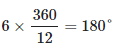2. A clock is started at noon. By 10 minutes past 5, the hour hand has turned through

A. 155°

B. 145°

C. 152°

D. 140°

Explanation: We know that angle traced by hour hand in 12 hrs = 360°
Time duration from noon to 10 minutes past 5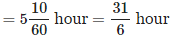Hence the angle traced by hour hand from noon to 10 minutes past 5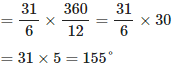3. At what time between 7 and 8 o'clock will the hands of a clock be in the same straight line but not together?

A. 5 minutes past 7

B.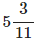minutes past 7

C.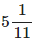minutes past 7

D.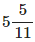minutes past 7

Explanation:

Solution 1

The two hands of a clock will be in the same straight line but not together between H and (H+1) o'clock at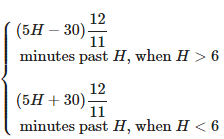Here H = 7.
Hands of the clock will point in opposite directions at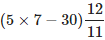minutes past 7
=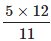minutes past 7
=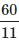minutes past 7
=minutes past 7

Solution 2

It's better to use formula as it can save lots of time in exams. However, we should understand the basics for sure. Please find the method given below to solve the same problem in the traditional way.
If the hands of the clock are in the same straight line, but not together, they will be 30-minute spaces apart.
At 7'o clock, the hands of the clock are 25-minute spaces apart. Hence the minute hand should gain 5-minute spaces over the hour hand so that the hands will be 30-minute spaces apart.
In 60 minutes, the minute hand gains 55-minute spaces over the hour hand.
Hence, to gain 5-minute spaces for the minute hand, the time needed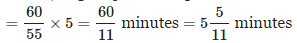That means when the time isminutes past 7, the hands of a clock will be in the same straight line but not together.

4. At what time between 5.30 and 6 will the hands of a clock be at right angles?

A. 44 minutes past 5

B.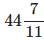minutes past 5

C.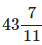minutes past 5

D. 43 minutes past 5

Explanation:

Solution 1

The two hands of the clock will be at right angles between H and (H+1) o' clock at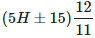minutes past H 'o clock

Let's see the times at which right angles are formed between 5 and 6
Let's take H=5. Hence the two hands will be at right angles between 5 and 6 at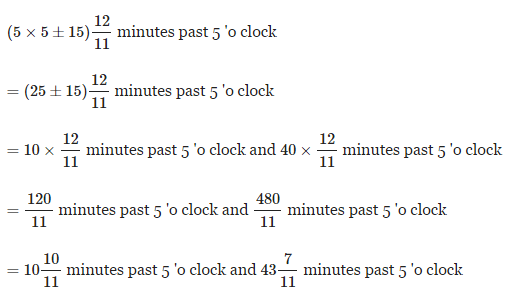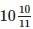minutes past 5 comes before 5.30.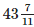minutes past 5 comes between 5.30 and 6. The question is to find out the time between 5.30 and 6 when the hands of a clock will be at right angles. Hence the required time isminutes past 5

Solution 2

At 5, the hands are 25 minutes spaces apart.
To get a right angle when the time is between 5.30 and 6, the minute hand has to gain (25 + 15) = 40-minute spaces over the hour hand.
In 60 minutes, the minute hand gains 55-minute spaces over the hour hand.
Hence, to gain 40-minute spaces for the minute hand, the time needed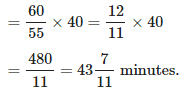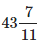That means when the time isminutes past 5, the hands of a clock will be at right angles.

5. At what angle the hands of a clock are inclined at 15 minutes past 5?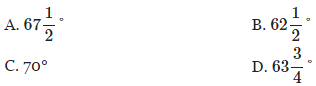Explanation:

Solution 1

The angle between hands of a clock
When the minute hand is behind the hour hand, the angle between the two hands at M minutes past H 'o clock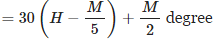When the minute hand is ahead of the hour hand, the angle between the two hands at M minutes past H 'o clock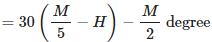Here H = 5, M = 15 and the minute hand is behind the hour hand.
Hence the angle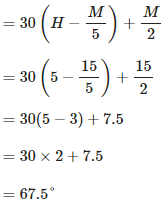Solution 2

15 minutes past 5
= 5 hour 15 minutes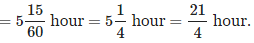Angle traced by hour hand in 12 hours = 360°
Hence angle traced by hour hand in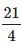hour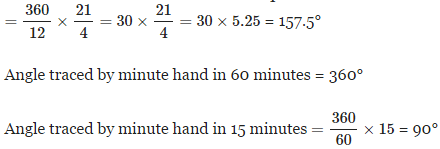Required angle = 157.5 - 90 = 67.5°

6. At 3:40, the hour hand and the minute hand of a clock form an angle of

A. 135°

B. 130°

C. 120°

D. 125°

Explanation:

Solution 1

The angle between hands of a clock
When the minute hand is behind the hour hand, the angle between the two hands at MM minutes past HH 'o clock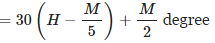When the minute hand is ahead of the hour hand, the angle between the two hands at MM minutes past HH 'o clockHere H = 3, M = 40 and the minute hand is ahead of the hour hand.
Hence the angle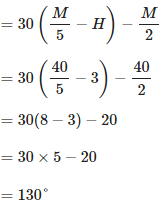Solution 2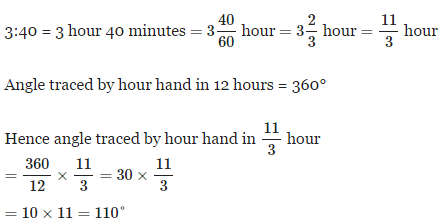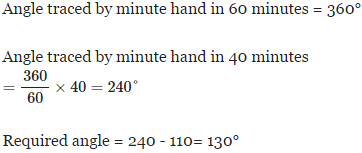The document Clocks based Questions - Notes | Study CSAT Preparation for UPSC CSE - UPSC is a part of the UPSC Course CSAT Preparation for UPSC CSE.
All you need of UPSC at this link: UPSC

## CSAT Preparation for UPSC CSE

72 videos|64 docs|92 tests
 Use Code STAYHOME200 and get INR 200 additional OFF

## CSAT Preparation for UPSC CSE

72 videos|64 docs|92 tests

### How to Prepare for UPSC

Read our guide to prepare for UPSC which is created by Toppers & the best Teachers

Track your progress, build streaks, highlight & save important lessons and more!

,

,

,

,

,

,

,

,

,

,

,

,

,

,

,

,

,

,

,

,

,

;